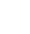# Maths

### Overview

"Pure mathematics is, in its way, the poetry of logical ideas."
Albert Einstein

At The Swanage School we want to provide students with the ability to reason mathematically and appreciate the beauty and power of mathematics. Mathematics is a vehicle for understanding the world. It is essential to everyday life, critical to science, technology and engineering, and necessary for financial literacy and most forms of employment. We aim to give students a sense of enjoyment and curiosity about the subject as well as the qualification they need for the next steps in their life.

### Curriculum

#### Key Stage 3

In Year 7 and 8 we follow the national guidance for Key Stage 3. The national curriculum for mathematics aims to ensure that all students:

• become fluent in the fundamentals of mathematics, including the ability to recall and apply knowledge rapidly and accurately
• reason mathematically by following a thought process and developing an argument, justification or proof using mathematical language
• can solve problems by applying their mathematics to a variety of problems with increasing sophistication

Each half term we cover 2 or 3 topics from our 6 main areas of maths and monitor student progress through assessment activities such as rich task investigations, challenge questions, topic tests and home learning tasks. The six areas are:

• Number (place value, decimals, types of numbers, factors and multiples)
• Algebra (algebraic notation, expanding brackets and solving equations)
• Ratio, proportion and rates of change (fractions, percentages, scale and converting between units)
• Geometry (angles, area and perimeter and 3D shapes)
• Probability (record, describe and analyse the frequency of outcomes)
• Statistics (collecting and representing data)

#### Year 9 and Key Stage 4 (Year 10 and 11)

During Year 9, 10 and 11 students will focus on the GCSE Mathematics course. This is designed to:

• develop fluent knowledge, skills and understanding of mathematical methods and concepts
• acquire, select and apply mathematical techniques to solve problems
• reason mathematically, make deductions and inferences and draw conclusions
• comprehend, interpret and communicate mathematical information in a variety of forms appropriate to the information and context

Over the course students will study maths looking at number, algebra, geometry, measures, probability and statistics, which continue learning from the six main areas of maths outlined above.

For the exams, students take three written papers, either all at Foundation or all at Higher level.

Students with particular mathematical aptitude may also study Further Maths as a Level 2 Certificate, which bridges the gap nicely between GCSE and A-level.

### Further information

Exam Board: AQA Mathematics